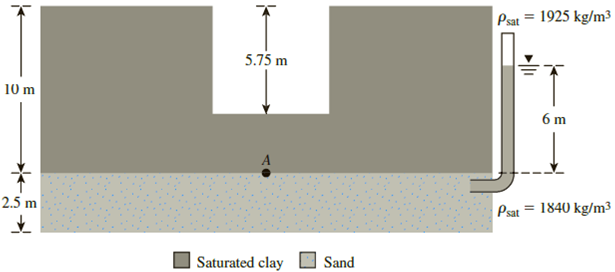Chapter 9, Problem 9.10PPrinciples of Geotechnical Enginee...

9th Edition
Braja M. Das + 1 other
ISBN: 9781305970939

Solutions

Chapter
SectionPrinciples of Geotechnical Enginee...

9th Edition
Braja M. Das + 1 other
ISBN: 9781305970939
Textbook Problem

Refer to Problem 9.9. Water may be introduced into the cut to improve the stability against heaving. Assuming that a cut is made up to the maximum permissible depth calculated in Problem 9.9, what would be the required height of water inside the cut in order to ensure a factor of safety of 1.5?9.9 Refer to Figure 9.28. What would be the maximum permissible depth of cut before heaving would occur?Figure 9.28

To determine

Find the required height (h) of water inside the cut in order to ensure a factor of safety of 1.5.

Explanation

Given information:

The thickness h1 of the stiff saturated clay is 10 m.

The depth h2 of the cut is 5.75 m.

The depth (d) of water table is 6.0 m.

The density of the saturated clay ρsat(clay) is 1,925kg/m3.

The density of the sand ρsand is 1,840kg/m3.

The maximum permissible depth (H) is 6.88 m.

The factor of safety is 1.5.

Calculation:

Consider the stability of point A in terms of heaving:

Calculate the saturated unit weight γsat(clay) of the clay using the relation.

γsat(clay)=ρsat(clay)γwaterρwater

Here, γwater is the unit weight of the water and ρwater is the density of water.

Take the unit weight of the water as 9.81kN/m3.

Take the density of the water as 1,000kg/m3

Substitute 1,925kg/m3 for ρsat(clay), 9.81kN/m3 for γwater, and 1,000kg/m3 for ρwater.

γsat(clay)=1,925(9.81)1,000=18.88kN/m3

Calculate the total stress σA at point A using the relation.

σA=(h1H)γsat(clay)+γw×h

Substitute 10 m for h1, 6

Still sussing out bartleby?

Check out a sample textbook solution.

See a sample solution

The Solution to Your Study Problems

Bartleby provides explanations to thousands of textbook problems written by our experts, many with advanced degrees!

Get Started

What is a relationship, and what three types of relationships exist?

Database Systems: Design, Implementation, & Management

Describe ways that team members collaborate.

Enhanced Discovering Computers 2017 (Shelly Cashman Series) (MindTap Course List)

How does an injector-type mixing chamber work?

Welding: Principles and Applications (MindTap Course List)